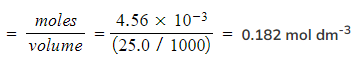# Edexcel A Level Chemistry:复习笔记4.1.3 Determining Concentrations

### Core Practical 3: Hydrochloric Acid Concentration

#### Performing the Titration

• The key piece of equipment used in the titration is the burette
• Burettes are usually marked to a precision of 0.10 cm3
• Since they are analogue instruments, the uncertainty is recorded to half the smallest marking, in other words to ±0.05 cm3

• The end point or equivalence point occurs when the two solutions have reacted completely and is shown with the use of an indicator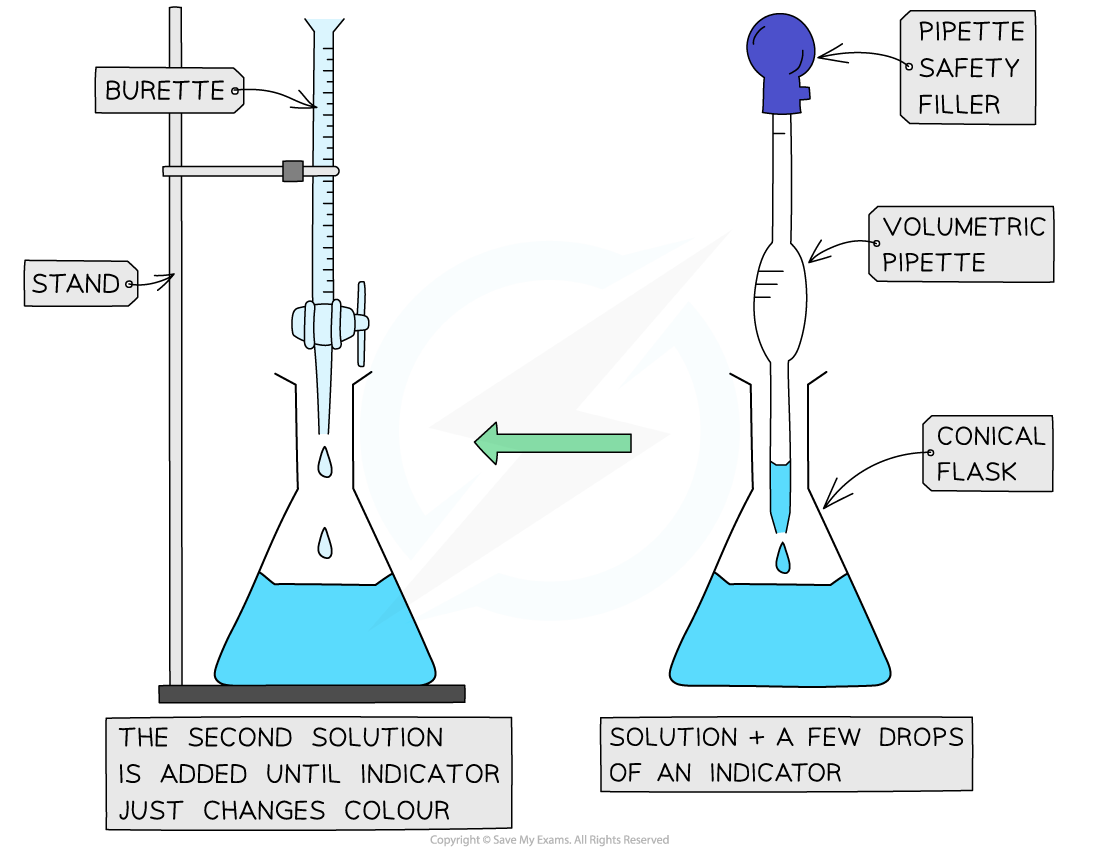The steps in a titration

• A white tile is placed under the conical flask while the titration is performed, to make it easier to see the colour change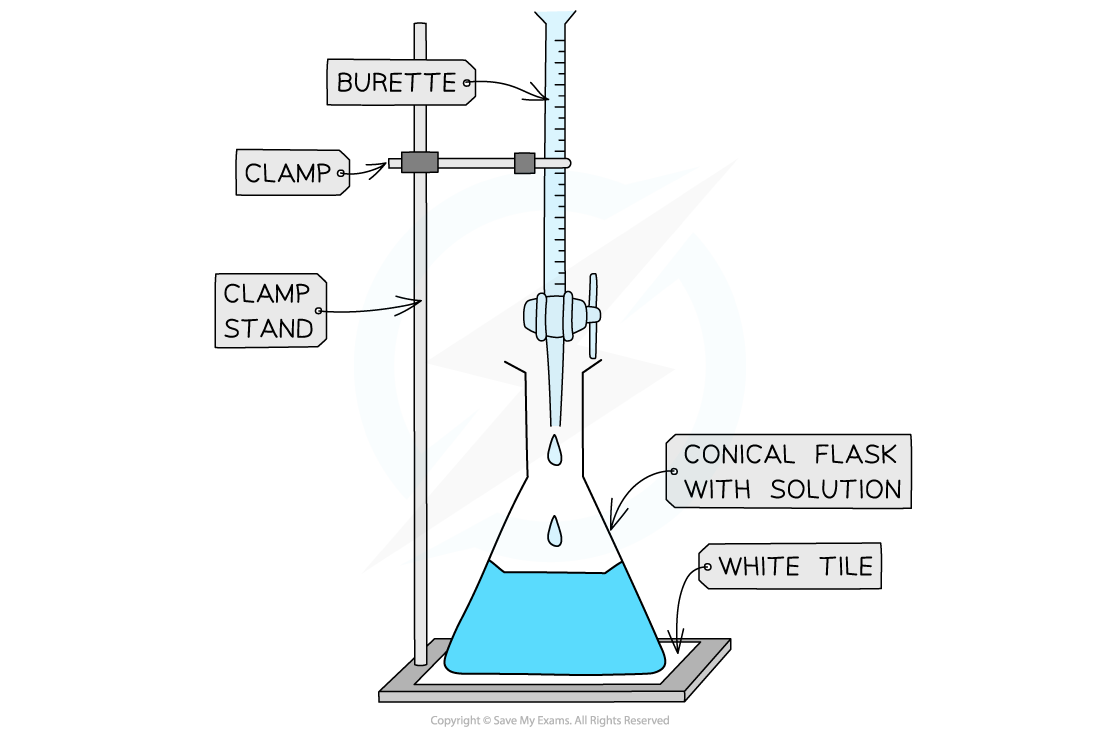Titrating

• The steps in a titration are:
• Measuring a known volume (usually 20 or 25 cm3) of one of the solutions with a volumetric pipette and placing it into a conical flask
• The other solution is placed in the burette
• To start with, the burette will usually be filled to 0.00 cm3

• A few drops of the indicator are added to the solution in the conical flask
• The tap on the burette is carefully opened and the solution added, portion by portion, to the conical flask until the indicator starts to change colour
• As you start getting near to the end point, the flow of the burette should be slowed right down so that the solution is added dropwise
• You should be able to close the tap on the burette after one drop has caused the colour change

• Multiple runs are carried out until concordant results are obtained
• Concordant results are within 0.1 cm3 of each other

#### Recording and processing titration results

• Both the initial and final burette readings should be recorded and shown to a precision of  ±0.05 cm3, the same as the uncertainty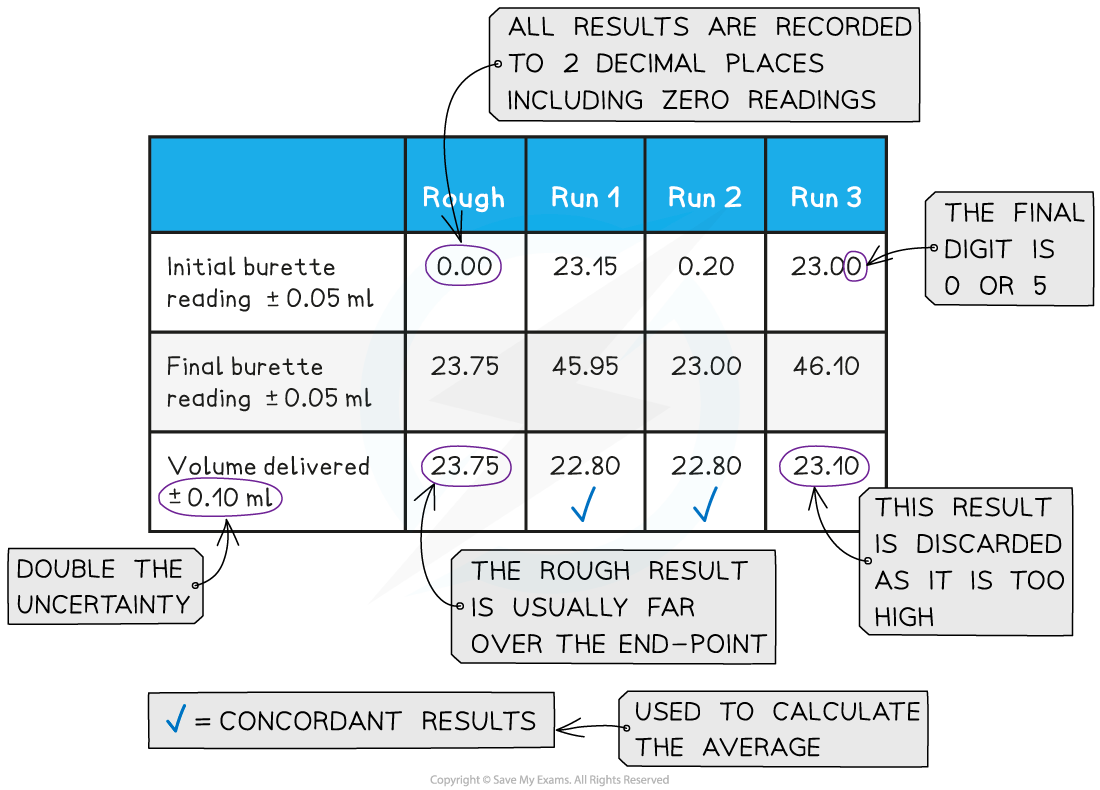A typical layout and set of titration results

• The volume delivered (titre) is calculated and recorded to an uncertainty of ±0.10 cm3
• The uncertainty is doubled, because two burette readings are made to obtain the titre (V final – V initial), following the rules for propagation of uncertainties

• Concordant results are then averaged, and non-concordant results are discarded
• The appropriate calculations are then done

#### Worked Example

25.0 cm3 of hydrochloric acid was titrated with a 0.200 mol dm-3 solution of sodium hydrogencarbonate, NaHCO3.

NaHCO3 + HCl → NaCl + H2O + CO2

Use the following results to calculate the concentration of the acid, to 3 significant figures.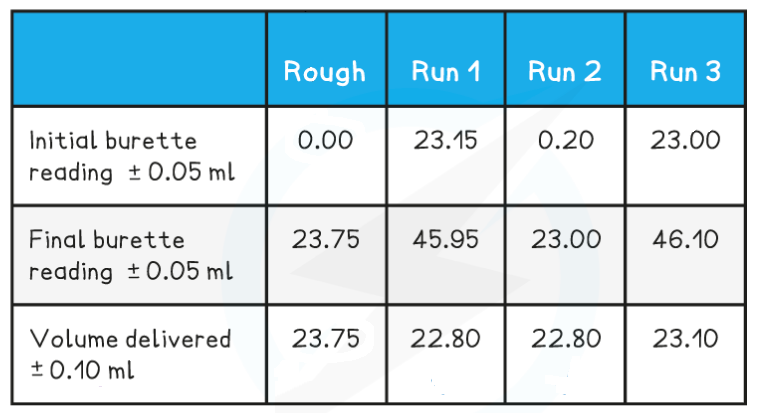Step 1: Calculate the average titre

• Average titre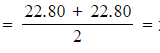22.80 cm3

Step 2: Calculate the number of moles of sodium hydrogencarbonate

• Moles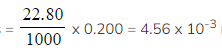moles

Step 3: Calculate (or deduce) the number of moles of hydrochloric acid

• The stoichiometry of NaHCO3 : HCl is 1 : 1
• Therefore, the number of moles of sodium hydrogencarbonate is also 4.56 x 10-3 moles

Step 4: Calculate the concentration of hydrochloric acid

• Concentration